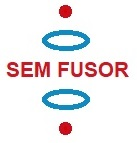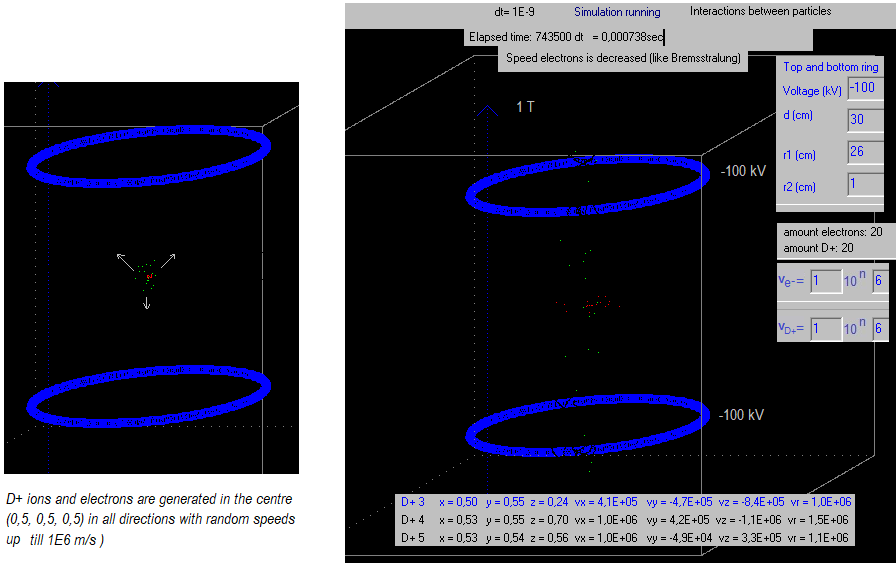A new idea to obtain clean fusion energy

16. Confinement of positive ions and electrons with a static electric and magnetic field

Inject of shoot from both sides deuterium into the SEM fusor

Back to main page

 The idea is that we shoot a kind of neutral beam with deuterium from top to bottom and from bottom to top. Another idea is to shoot a kind of capsula with deuterium from the top and from the bottom towards the centre. In the centre they collide, the beams or the capsulas, and it would be as if the ions and electrons are generated in the centre. Below is a simulation where we generate D+ ions and electrons in the centre     http://fusionwiki.ciemat.es/wiki/TJ-II:Neutral_Beam_Injection   -> Here neutral hydrogen is accelerated to 40 keV -> 40.103 . 1.6×10−19 J = 6,4.10-15 J = 1.m.v2       mhydrogen = 1,67.10-27 kg   -> v = √ ( 3,8.1012   ) =1,96 106 m/s This is about the same speed as we generate the particles in our simulation program.   https://en.wikipedia.org/wiki/Migma ( Migma uses self-intersecting beams of ions from small particle accelerators to force the ions to fuse)     Fig.1. Exp. 16.1ve:=2*vie; electron[i].vx:=- 0.5*ve+ ve*random; {so the maximum speed is vie} electron[i].vy:= -0.5*ve+ ve*random; {random -> 0 <= X < 1 } electron[i].vz:= -0.5*ve + ve*random; electron[i].x:=0.50+ ( - 0.5 + random) /100; {so they start not in exactly the same point} electron[i].y:=0.50 + ( - 0.5 + random) /100; {so they start not in exactly the same point} electron[i].z:= 0.50 + ( - 0.5 + random) /100; //0.35+ ( - 0.5 + random) /100; {so they start not in exactly the same point}   The speeds of the electrons are decreased in the program, to simulate Bremsstrahlung.   if (vx>1E1) and (vy>1E1) and (vz>1E1)  {decreasing until one of the speed components is already small} then Begin vx:=vx*0.90; vy:=vy*0.90; vz:=vz*0.90; end;         Fig. 2 (from Wikipedia)       What we want in the Sem Fusor is a bunch of D+ ions and electrons confined, the D+ ions moving up and down and the electrons forming a negative electron cloud in the centre. Questions: Is it necessary to neutralize the D+ ions to make a neutral beam, or is it better to inject the D+ ions without neutralizing? Inject the D+ ions and electrons from both sides, or only from one side? If we inject a neutral beam, for the top and from the bottom, will D+ ions be formed in the centre? (will the neutral beams collide in the centre?). Will fusion occur? What will be the losses?   It should be interesting to do real systematic experiments.20 February 2014 ..     by  Rinze Joustra        www.valgetal.com